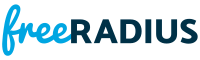rlm_eap_sim.c File Reference
#include <stdio.h>
#include <stdlib.h>
#include "../../eap.h"
#include "eap_types.h"
#include "eap_sim.h"
#include "comp128.h"Include dependency graph for rlm_eap_sim.c:

Go to the source code of this file.

Data Structures

struct  eap_sim_server_state

Macros

#define EAPTLS_MPPE_KEY_LEN   32

Typedefs

typedef struct eap_sim_server_state eap_sim_state_t

Functions

static int CC_HINT (nonnull)

static int eap_sim_compose (eap_session_t *eap_session)

static int eap_sim_get_challenge (eap_session_t *eap_session, VALUE_PAIR *vps, int idx, eap_sim_state_t *ess)

static int eap_sim_sendchallenge (eap_session_t *eap_session)
Send the challenge itself. More...

static int eap_sim_sendstart (eap_session_t *eap_session)

static int eap_sim_sendsuccess (eap_session_t *eap_session)

static void eap_sim_stateenter (eap_session_t *eap_session, eap_sim_state_t *ess, enum eapsim_serverstates newstate)
Run the server state machine. More...

static int mod_process (UNUSED void *arg, eap_session_t *eap_session)
Authenticate a previously sent challenge. More...

static int process_eap_sim_challenge (eap_session_t *eap_session, VALUE_PAIR *vps)
Process an EAP-Sim/Response/Challenge. More...

static int process_eap_sim_start (eap_session_t *eap_session, VALUE_PAIR *vps)
Process an EAP-Sim/Response/Start. More...

Variables

rlm_eap_module_t rlm_eap_sim

Data Structure Documentation

 struct eap_sim_server_state

Definition at line 40 of file rlm_eap_sim.c.Collaboration diagram for eap_sim_server_state:
Data Fields
struct eapsim_keys keys
int sim_id
enum eapsim_serverstates state

Macro Definition Documentation

 #define EAPTLS_MPPE_KEY_LEN   32

Definition at line 370 of file rlm_eap_sim.c.

Typedef Documentation

 typedef struct eap_sim_server_state eap_sim_state_t

Function Documentation

 static int CC_HINT ( nonnull )
static

Definition at line 448 of file rlm_eap_sim.c.Here is the call graph for this function:

 static int eap_sim_compose ( eap_session_t * eap_session )
static

Definition at line 49 of file rlm_eap_sim.c.Here is the call graph for this function:Here is the caller graph for this function:

 static int eap_sim_get_challenge ( eap_session_t * eap_session, VALUE_PAIR * vps, int idx, eap_sim_state_t * ess )
static

Definition at line 116 of file rlm_eap_sim.c.Here is the call graph for this function:Here is the caller graph for this function:

 static int eap_sim_sendchallenge ( eap_session_t * eap_session )
static

Send the challenge itself.

Challenges will come from one of three places eventually:

1 from attributes like PW_EAP_SIM_RANDx (these might be retrieved from a database)

2 from internally implemented SIM authenticators (a simple one based upon XOR will be provided)

3 from some kind of SS7 interface.

For now, they only come from attributes. It might be that the best way to do 2/3 will be with a different module to generate/calculate things.

Definition at line 266 of file rlm_eap_sim.c.Here is the call graph for this function:Here is the caller graph for this function:

 static int eap_sim_sendstart ( eap_session_t * eap_session )
static

Definition at line 58 of file rlm_eap_sim.c.Here is the call graph for this function:Here is the caller graph for this function:

 static int eap_sim_sendsuccess ( eap_session_t * eap_session )
static

Definition at line 380 of file rlm_eap_sim.c.Here is the call graph for this function:Here is the caller graph for this function:

 static void eap_sim_stateenter ( eap_session_t * eap_session, eap_sim_state_t * ess, enum eapsim_serverstates newstate )
static

Run the server state machine.

Definition at line 408 of file rlm_eap_sim.c.Here is the call graph for this function:Here is the caller graph for this function:

 static int mod_process ( UNUSED void * arg, eap_session_t * eap_session )
static

Authenticate a previously sent challenge.

Definition at line 607 of file rlm_eap_sim.c.Here is the call graph for this function:Here is the caller graph for this function:

 static int process_eap_sim_challenge ( eap_session_t * eap_session, VALUE_PAIR * vps )
static

Process an EAP-Sim/Response/Challenge.

Verify that MAC that we received matches what we would have calculated from the packet with the SRESx appended.

Definition at line 557 of file rlm_eap_sim.c.Here is the call graph for this function:Here is the caller graph for this function:

 static int process_eap_sim_start ( eap_session_t * eap_session, VALUE_PAIR * vps )
static

Process an EAP-Sim/Response/Start.

Verify that client chose a version, and provided a NONCE_MT, and if so, then change states to challenge, and send the new challenge, else, resend the Request/Start.

Definition at line 497 of file rlm_eap_sim.c.Here is the call graph for this function:Here is the caller graph for this function:

Variable Documentation

 rlm_eap_module_t rlm_eap_sim
Initial value:
= {
.name = "eap_sim",
.session_init = mod_session_init,
.process = mod_process,
}
static int mod_session_init(void *instance, eap_session_t *eap_session)
Definition: rlm_eap_gtc.c:89
static int mod_process(UNUSED void *arg, eap_session_t *eap_session)
Authenticate a previously sent challenge.
Definition: rlm_eap_sim.c:607

Definition at line 690 of file rlm_eap_sim.c.